# Correlation Processor

Modified on Tue, 30 Nov 2021 at 11:34 AM

# Overview

The correlation processor computes the correlation matrix for a given input data set.

A correlation matrix is a table showing correlation (dependence) coefficients between variables. Each cell in the table shows the correlation between two variables. A correlation matrix is used to summarize data, as an input into a more advanced analysis, and as a diagnostic for advanced analyses. Further information about the Correlation matrix can be found in the following link.

# Input

The input Dataset should contain columns (at least two) of type numeric, double or integer in order to compute the correlation between these variables.

# Configuration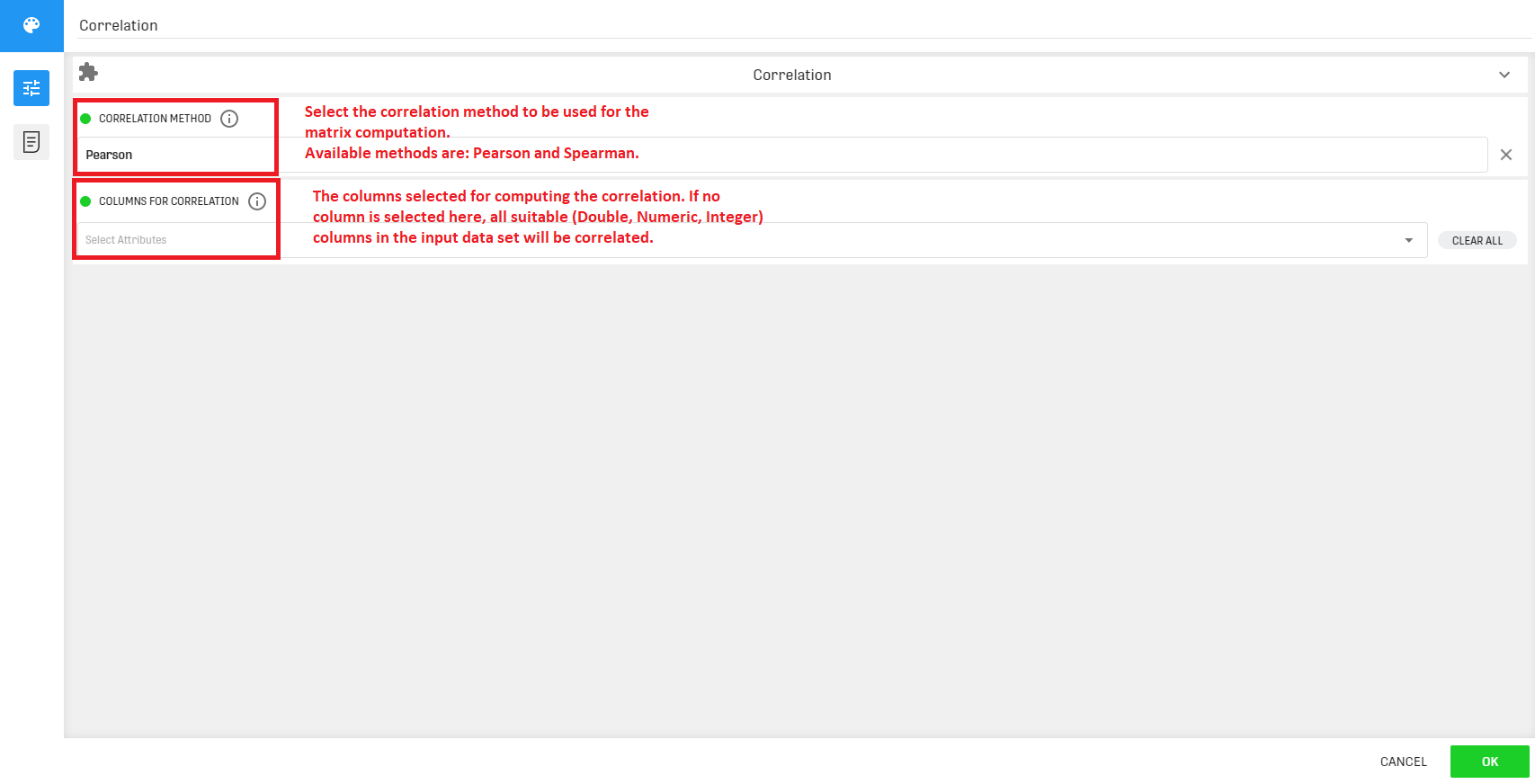The Pearson and Spearman Correlations are slightly different, while the Pearson correlation indicates the extent to which two variables are linearly related, the Spearman correlation is used on ordinal variables and indicates to what extent two variables are monotonously related. For further information about Pearson and Spearman correlations you can consult the following link.

# Output

The output correlation matrix is presented in two different ways:

• Within the correlation process: The output correlation matrix can be viewed as it is, selected columns are displayed along with the corresponding correlation values. A color feature is added where a red background implies a negative correlation and a green background implies a positive correlation.
• Within the result table: The correlation matrix is resized into a three-column table with the respective values: Column1, Column2 and the correlation between the two columns.

# Example

In this example we want to compute the Pearson correlation between selected numeric variables included in a dataset containing different companies along with corresponding relevant numbers (sales, profit..).

## Example Input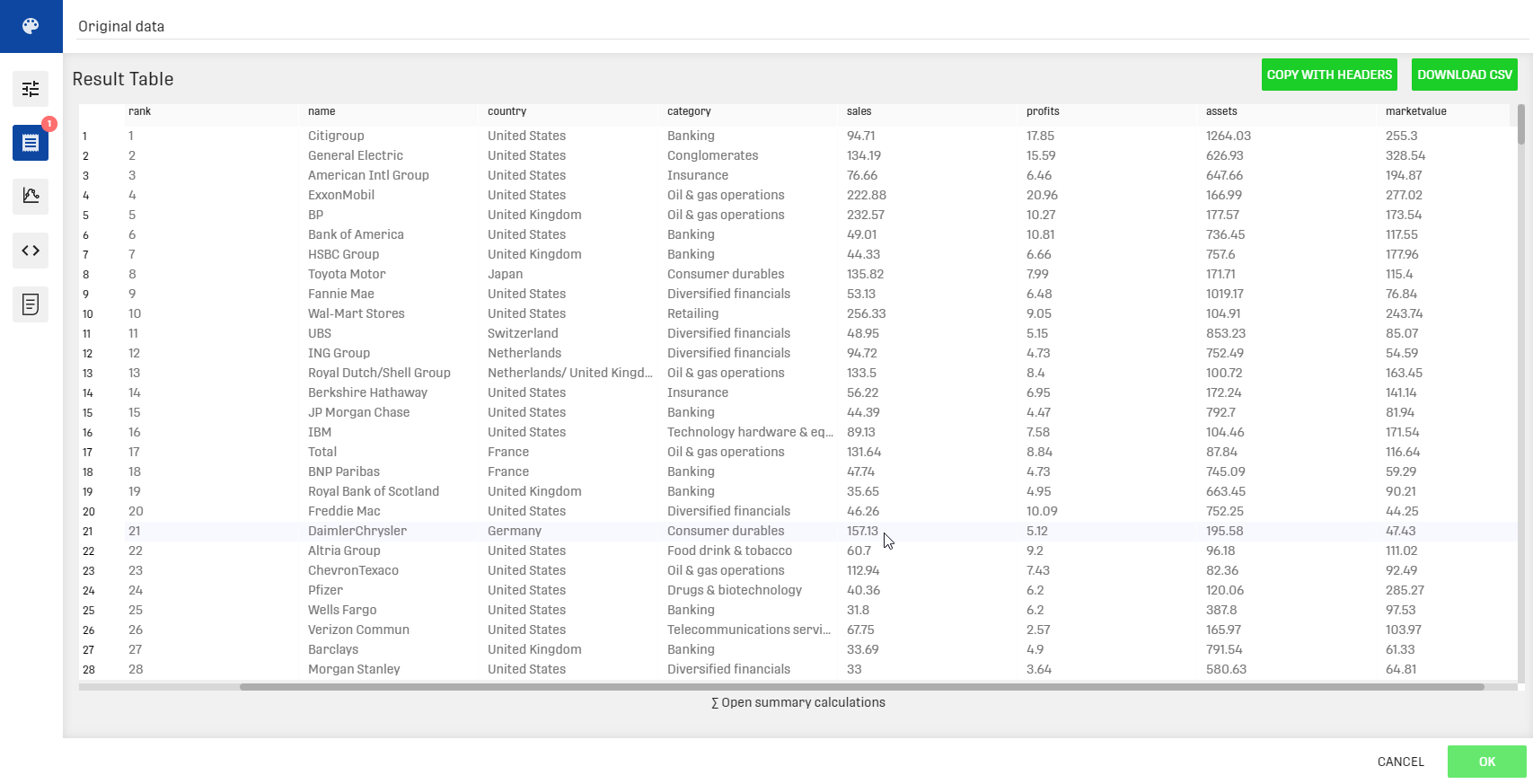## Workflow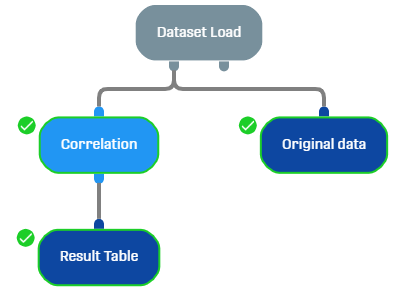## Example Configuration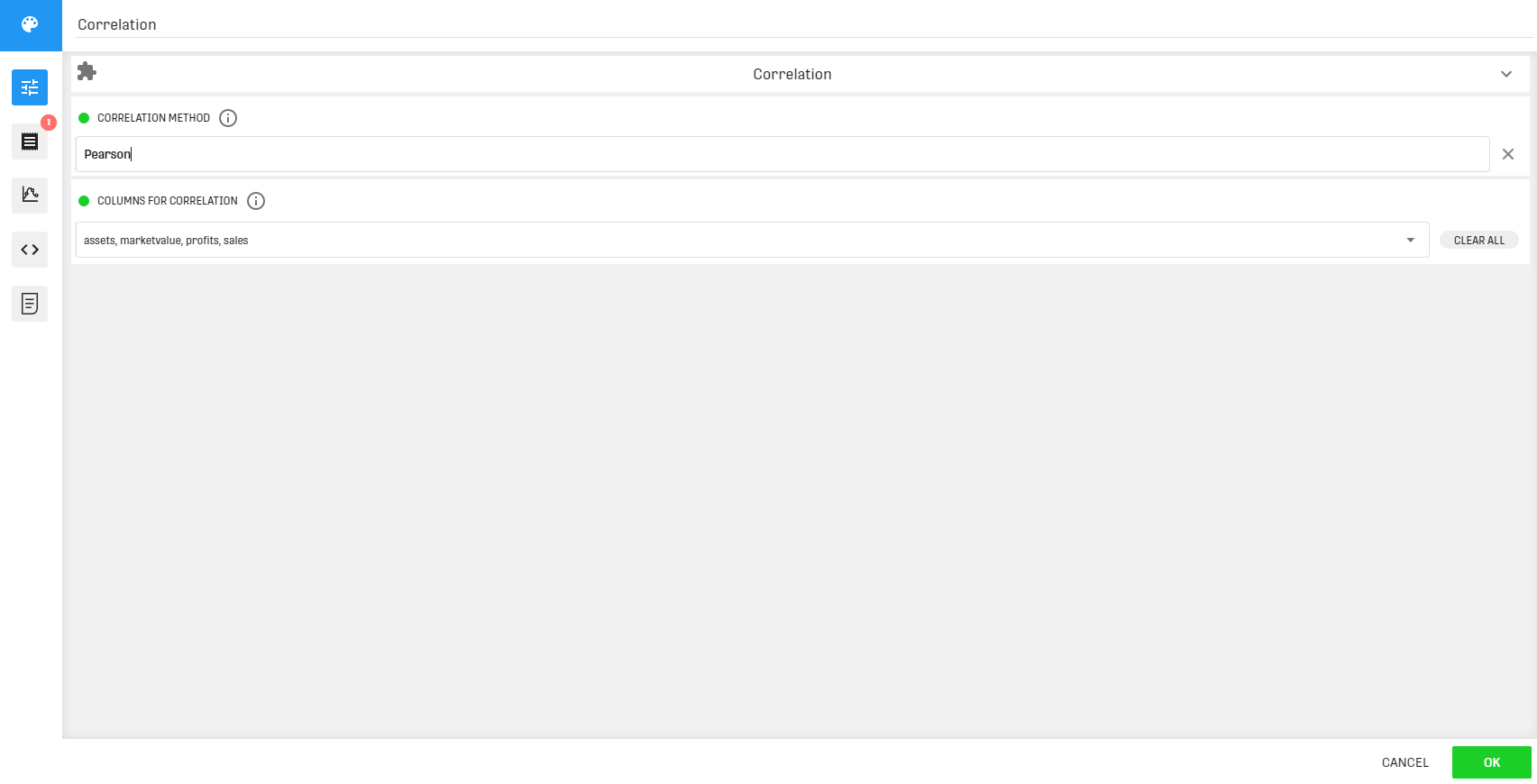We chose the Pearson correlation method and selected all columns containing numeric values.

## Result

The correlation matrix is accessible via the results tab within the correlation process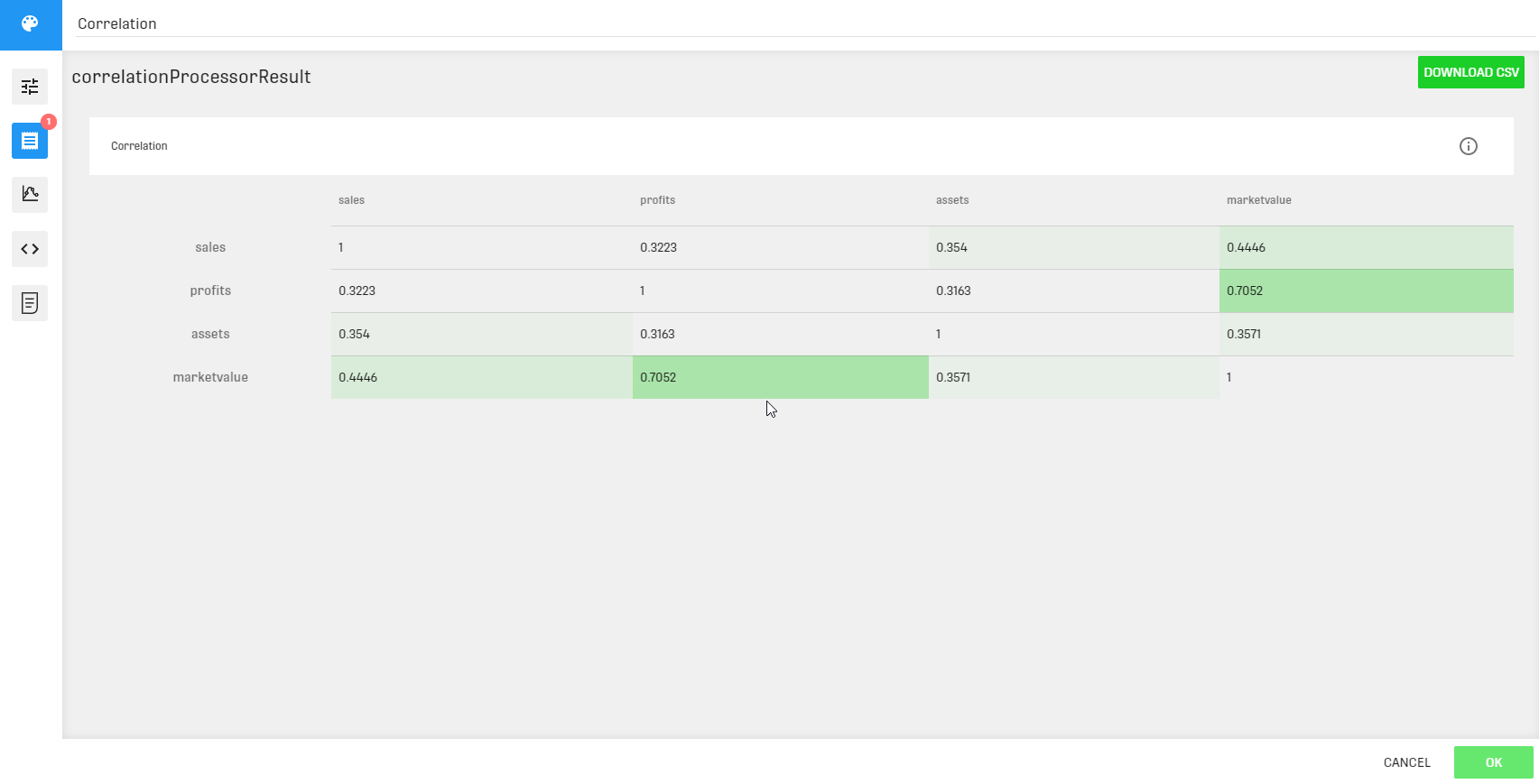The correlation matrix in this example contains only positive values. The corresponding background colors are different shades of green depending on the value. The most intense background color is associated to the correlation value between "profits" and "marketvalue" which represents the highest correlation value in the matrix. (diagonal values are not included as they represent the similarity of two variables representing the same column).

The result table contains the resized correlation matrix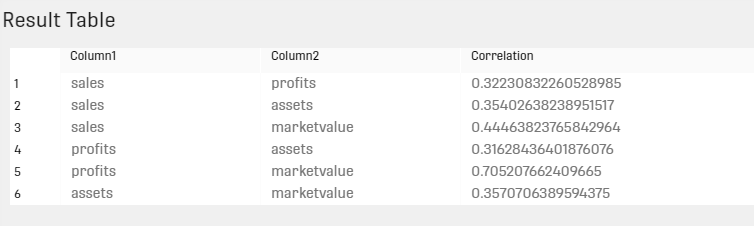The result table can be useful in case the difference in green can't be spotted. Using the result table, the correlation values can be easily sorted.

# Related Articles

Cross Correlation Processor